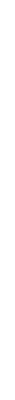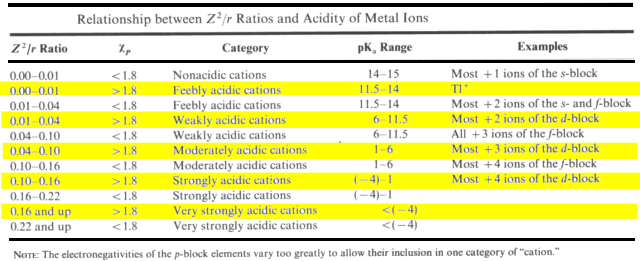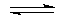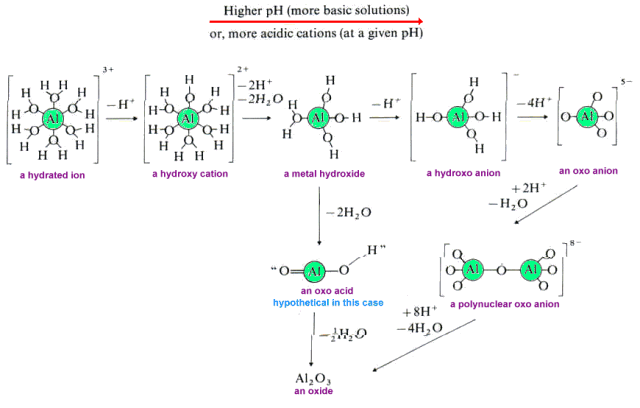## Predicting the Degree of Hydrolysis of Cations

It is useful to be able to gauge the approximate acidity of a given metal cation because it allows us to anticipate if a compound will react violently when exposed to water or atmospheric humidity, whether its hydroxide or oxide will precipitate on forming an aqueous solution or determine the form in which a metal ion pollutant exists in a lake, i.e., will it be an insoluble sludge at the bottom or will it remain in solution to be taken up by living organisms.

Although equations have been derived which accurately predict the extent of hydrolysis of metal ions, these are too complicated to be used for quick estimations of approximate acidity. Acidity of metal ions (their ability to produce hydronium ions in aqueous solution) is a function of their charge and size.

 pKa = 15.14 - 88.16(Z2/r)

A plot of pKa versus Z2/r shows a linear relationship. On the graph below, metals with electronegativities less than 1.5 are plotted as purple circles. Recall that the larger the value of pKa, the less acidic the cation. Click on the graph to see the details.### Electronegativity Effects

Cations of metals having electronegativities greater than 1.5 have smaller pKas (are more acidic) than other metal ions of similar charge and size. The equation can be modified to include the effect of "excess" electronegativity of a cation and it "excess" acidity.

 pKa = 15.14 - 88.16[(Z2/r) + 0. 096(EN-1.50)]

This equation is only valid if the Pauling electronegativity of metal is greater than 1.50

A plot of pKa versus [(Z2/r) + 0.096 (EN-1.50)] shows a linear relationship. On the graph below, metals with electronegativities less than 1.5 are plotted as open circles. Click to see the details.Taken from Wulfsberg, Gary. Principles of Descriptive Chemistry; Brooks/Cole Publishing:Monterey CA, 1987; p.29.

 PROBLEMS Classify the following cations and describe their reactions with water.Eu2+ B3+ W6+

### Halides That Fail to Undergo Hydrolysis

Some elements which would be expected to react explosively with water are inert.

Carbon tetrachloride is an example. Although Z2/r is 0.533, CCl4 insoluble and non-reactive in water. The fact that the C4+ ion is so small is the key. There is a limit (the maximum coordination number) to the number of atoms that may surround it. As the radius of the ion increases, the maximum coordination number also increases. Maximum coordination number increases down a family in the periodic table.

Period 2 elements have a maximum coordination number of 4 (the basis of the octet rule). Elements in Periods 3 & 4 can bond with as many a six atoms. Elements in Periods 5 & 6 can have even larger coordination numbers. For some ions in the middle of Periods 5 & 6 such as Ag+, Hg2+, Au+, Sn2+ the preferred coordination number is low, often two. This affects the acidities of these cations. Hg2+ and Sn2+ have aciditiies that are higher than we would calculate from the pKa formula due to the fact that there are fewer water molecules coordinated to the metal ion causing a stronger electrostatic force pulling on the electrons of each water molecule. Be2+, the smallest hydrated ion, is also more acidic than we would calculate.

### Species Formed During Cation Hydrolysis

When a water molecule is ionized upon coordination to a metal ion, a hydroxide group is left attached to the metal.

[M(H2O)n]z+ + H2O[M(H2O)n-1(OH)](z-1)+ + H3O+

This is only the first of several reactions that can occur. Additional coordinated water molecules may also hydrolyze. The resulting metal cations which possess multiple hydroxy groups are called hydroxo cations. The hydroxo cation may undergo a variety of chemical reactions. Let's use the hydrolysis of Al3+ as an example.For most metal cations the most significant reactions are generating hydroxides that are often insoluble.

z[M (aq)(OH)](z-1)M(OH)3 (s) + (z-1)[M(aq)]z+

For many metal cations, Keq for this reaction is approximately 106.5. Therefore the equilibrium lies to the right precipitating metal hydroxides unless the solution is sufficiently acidic to react reprotonate the hydroxo cation. In the case of Al3+, the hydroxo cation produces a gelatinous precipitate of aluminum hydroxide.

z[Al (aq)(OH)](z-1)Al(OH)3 (s) + (z-1)[Al(aq)]z+

The pH at which the metal hydroxide precipitates can be determined by the equation:In most cases, this pH value is roughly equal to the pKa value of the metal cation. Thus, the lower the pKa value for a metal ion, the less basic the solution needs to be for precipitation of a metal hydroxide to begin. Although the concentration of the metal ion has some effect on the pH, moderately acidic ions such as Al3+ will form insoluble hydroxides even in solutions of low pH.

The insoluble metal hydroxides often lose molecules of water to yield insoluble metal oxides.

M(OH)3 (s)MOz/2 (s) + z/2 H2O

It is difficult to distinguish the metal hydroxide and oxide precipitates so we will not attempt to do so.

If the metal ion attracts the electrons shared with oxygen strongly enough, the hydroxide may act like a weak oxo acid ionizing to yield hydronium ions and an oxo anion.

M(OH)z + z H2OMOzz- + z H3O+